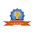# vs.eyeandcontacts.com

## Data, Data Handling and Probability Terms

Bar chart: A graph that uses parallel bars of equal width to display information.

Column: Items arranged in a vertical line.

Data: Numerical information that is used in statistics.

Frequency:
• The number of times a particular item appears in a set of data.
• The number of times that an event has occurred.

Graph: A drawing that shows a relationship between sets of data.

Mean: The average of a number of different amounts. To calculate the mean: sum of numbers ÷ number of numbers.

Median: When numbers are arranged from least to greatest, the middle number is the median.

Mode: The number that occurs most often in a set of numbers.

Pie chart: A circular diagram which is used to display data as sectors or sections of a circle (360º).

Range: The difference between the greatest and the least value in a set of data.

Certain event: An event that will definitely happen. A certain event has a probability of one.

Chance: The likelihood that a particular outcome will happen.

Equally likely: Events that have the same chance or probability.

Event: A possible outcome. Something that may happen.

Favourable outcome: In probability, the outcome you are interested in measuring.

Impossible event: An event that will definitely not happen. An event with a probability of zero.

Odds: The ratio of favourable outcomes to unfavourable outcomes.

Outcome: One of the possible results in a probability experiment.

Probability: The measure of how likely an event is. Its value lies between 0 (impossible event) and
1 (certain event).

Random selection: A chance pick from a number of items.

Tree diagram: A diagram shaped like a tree. Helps in showing possible outcomes of an event.

1.Anonymous12 July, 2021

Thanks for this post. I didn't knew about it.

1.2.Anonymous16 July, 2021

Good

1.HomeTop